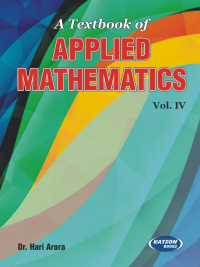•## A Textbook of Applied Mathmatics-IV

In Stock
ISBN 978-93-5014-684-2 Dr. Hari Arora S.K. Kataria & Sons 2019 In Stock
Rs 350.00 Rs 315.00

#### CONTENTS

Module-I: Iterative Techniques and Interpolation
• Solution of Algebraic and Transcendental Equation • Solution of Simultaneous Equation • Calculus of Finite Differences • Interpolation with Equal and Unequal Intervals
Module-II: Numerical Differentiation and Integration
• Numerical Differentiation and Integration • Numerical Solution at Ordinary Differential Equations
Module-III: Probability and Probability Distribution
• Probability and Probability Distribution
Module-IV: Dispersion, Curve Fitting and Principle of Least Square
• Measures of Dispersion • Curve Fitting and Principle of Least Squares
• Appendix
• Model Test Papers
• Index.

• Author : Dr. Hari Arora
• Publisher : S.K. Kataria & Sons
• Publishing Year : 2019